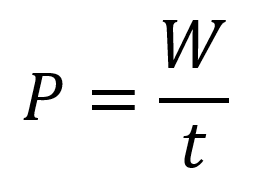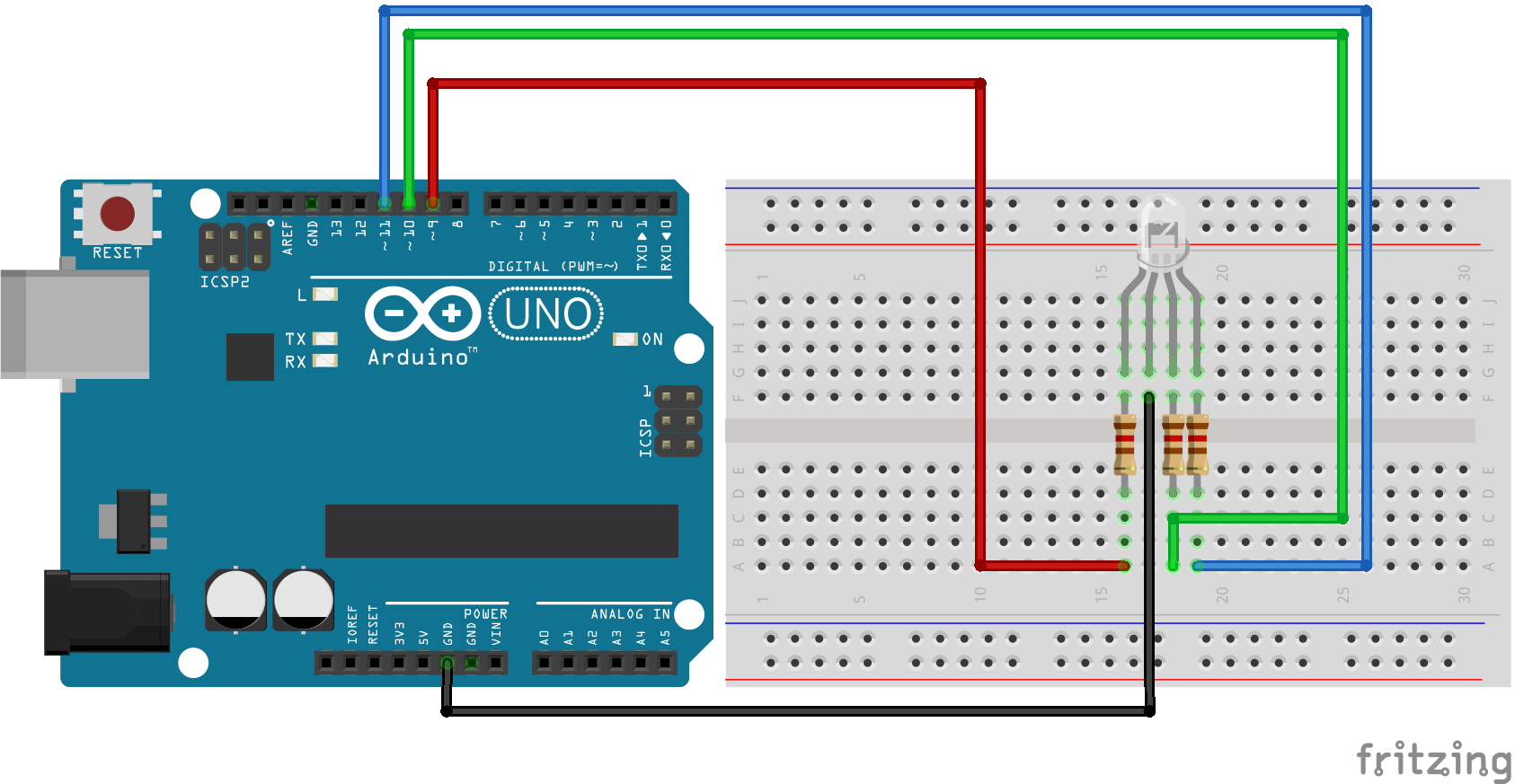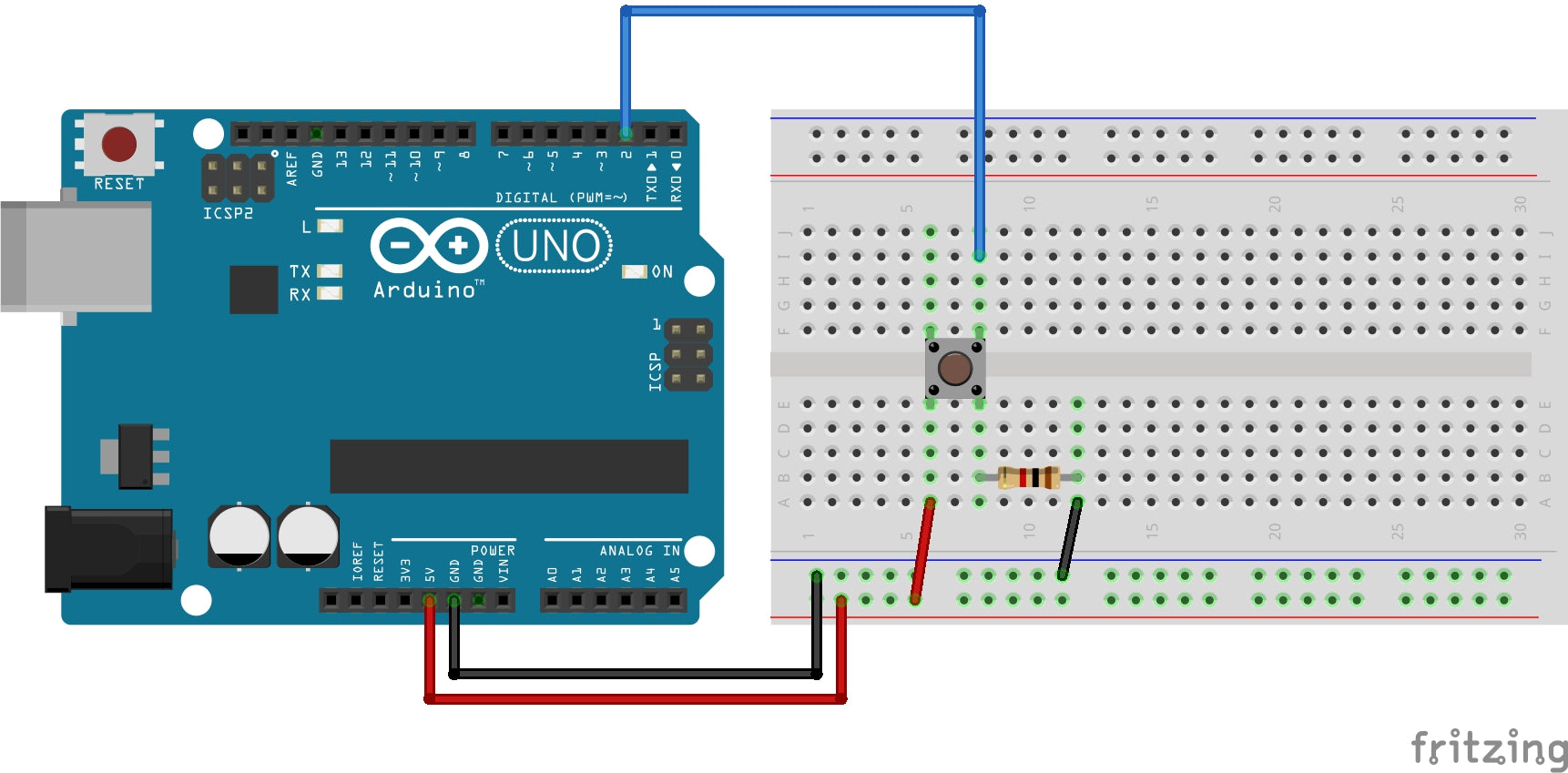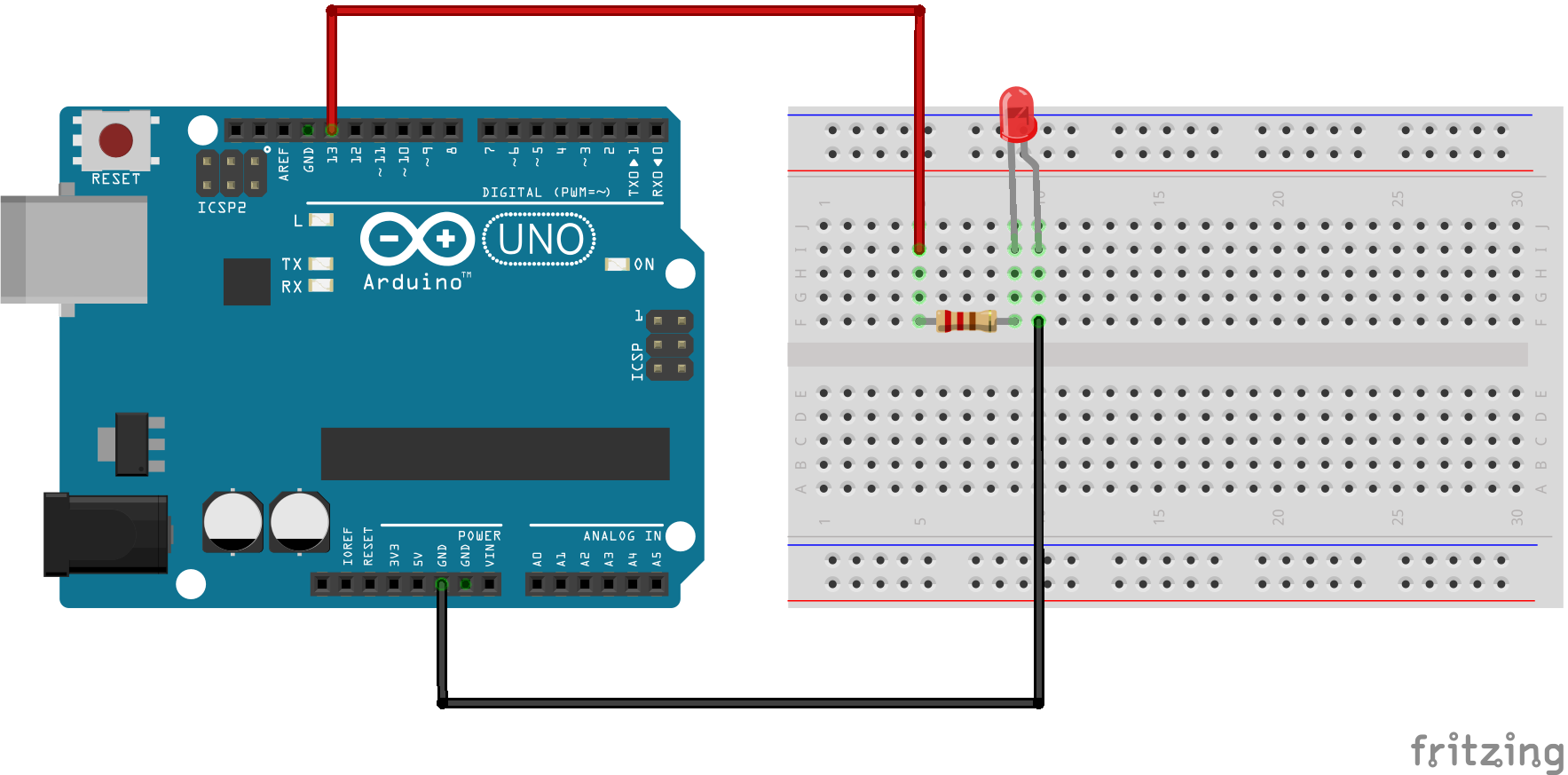# 0.0 Introduction

Energy is the ability to do work, and power is the rate at which energy is used.

Power ( $// $ ) is a certain amount of energy ( $// $ ) used in a certain length of time ( $// $ ), expressed as follows:

$// $

Where ( $// $ ) is power in watts (W), $// $ is energy is joules (J), and $// $ is time in seconds (s).

Note that an italic $// $ is used to represent energy in the form of work and a nonitalic W is used for watts, the unit of power. The joule (J) is the SI unit of energy.

Energy in joules divided by time in seconds gives power in watts. For example, if 50 J of energy are used in 2 s, the power is 50 J/2 s = 25W.

By definition,

One watt (W) is the amount of power when one joule of energy is used in one second.

# 1.0 Formula in Action

An amount of energy equal to 100 J is used in 5 s. What is the power in watts?

$// $

•### A Beginner's Guide to Connecting and Programmin...

RGB LEDs are versatile and dynamic lighting solutions that can display a wide range of colors. In this beginner-friendly guide, we'll show you how to connect and program an RGB...

### A Beginner's Guide to Connecting and Programmin...

RGB LEDs are versatile and dynamic lighting solutions that can display a wide range of colors. In this beginner-friendly guide, we'll show you how to connect and program an RGB...

•### Arduino Push Button

This example turns on the built-in LED on pin 13 when you press the button. 1.0 Background Knowledge 1.1 Push Buttons Pushbuttons or switches connect two points in a circuit...

### Arduino Push Button

This example turns on the built-in LED on pin 13 when you press the button. 1.0 Background Knowledge 1.1 Push Buttons Pushbuttons or switches connect two points in a circuit...

•### How to Blink an LED Using Arduino

This project is the "Hello World!" of Arduino. In this project, we are going to learn about physical outputs and two electronic components, while performing the simplest project in the...

### How to Blink an LED Using Arduino

This project is the "Hello World!" of Arduino. In this project, we are going to learn about physical outputs and two electronic components, while performing the simplest project in the...